Next: Logarithmic Functions Up: Trigonometric Functions Previous: Arctan

### Pi

The computation of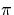has a long and colourful history (at least by mathematical standards), and has been much studied. The method chosen to implement an algorithm here is to use some identities due to the the mathematician John Machin in 1706. A fuller discussion of approaches to computingis available in .

Perhaps the simplest identity (by James Gregory and Gottfried Wilhelm Leibniz ) is: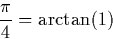However this identity is impractical because the sequence for arctan converges far too slowly with x=1 to be of value. Over 300 terms of the expansion of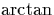given in section 5.4.1 would be required for two decimal places, and to achieve 100 correct digits would require a massive 1050 terms.

Machin's variation uses the tan double angle angle formula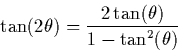to derive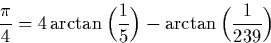There are other similar identities. The one used in this implementation is: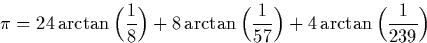These identities give a much faster of convergence because the sequence which approximatesis generated with inputs much closer to zero.

These identities are not the most effective method of computing.In fact more recent approaches allow the computation of an arbitrary hexadecimal digit ofwithout any computation of the preceding digits.Next: Logarithmic Functions Up: Trigonometric Functions Previous: Arctan
Martin Escardo
5/11/2000